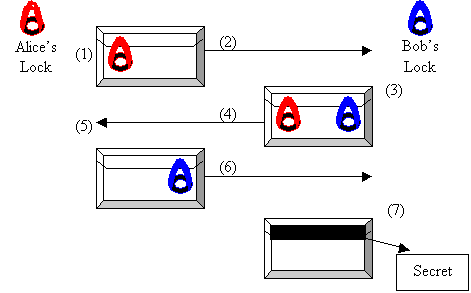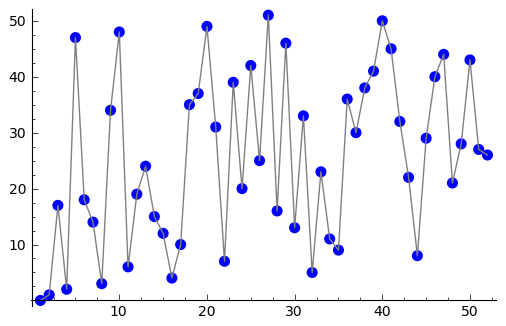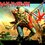# This note has been used to help create the Diffie-Hellman wiki

This the second installment in my series of post related to modern cryptography...Click here for part 1 on RSA ciphersIntroduction

$\textbf{Introduction:-}$Most ciphers require the sender and the receiver to exchange a key prior to exchanging coded messages.But the key which is exchanged may itself be vulnerable to attack by an unauthorized third party while it is being transmitted over a network.So serious effort is essential to ensure the safety the safety of the key so that it does not all into the wrong hands.key

$\textbf{An Analogy:-}$Suppose two people Alice and Bob want to exchange a secret.So at first Alice puts the secret in a box and locks it up using a padlock(which only she can unlock) and sends the locked box to Bob.Bob who is unable to open the padlock puts another padlock on the box(which only he can open and sends the box back to Alice.Now Alice receives the box with two padlocks and she opens up her padlock and returns the box to Bob.Notice that now the box only contains Bob's padlock.After receiving the box Bob opens up his own padlock and hence opens the box containing the secret.Imd

$\textbf{ALGORITHM(Diffie-Hellman):-}$

Step 1. Together Alice and Bob choose a 200-digit number p which is likely to be a prime and a number $g$ such that $1 < g

$\textit{Step 2.}$Alice secretly chooses an integer $n$

$\textit{Step 3.}$Bob secretly chooses an integer $m$

$\textit{Step 4.}$Alice computes $g^{n} \mod p$ and tells that to Bob.

$\textit{Step 5.}$Bob computes $g^{m} \mod p$ and tells that to Alice.

$\textit{Step 6.}$The shared secret key is now $s \equiv (g^{n})^{m} \equiv (g^{m})^{n} \equiv g^{mn} \pmod{p}$

Notice that both Alice and Bob can easily compute it. Alice computes this as $s \equiv (g^{m})^{n} \equiv g^{mn} \pmod{p}$ Bob computes this as $s \equiv (g^{n})^{m} \equiv g^{mn} \pmod{p}$

Now they can communicate with each other using their agreed upon secret key by encrypting messages using a suitable cipher(like Arcfour,Bowfish,Cast128 etc).

$\textbf{Safety and the Discreet Log problem:-}$ in order to understand the conversation between Alice and Bob the eavesdropper needs the shared secret k $s$.BUt it is extremely difficult to compute $s$ given only $p,g,g^{n} \mod p,g^{m} \mod p$.One way to compute would be to compute n from the knowledge of $g$ and $g^{n}$ bt for extremely large values of p this is computationally in-feasible.A polynomial time bound in a quantum computer was provided by Peter Shor.This is known as the $\textit{Discreet Logarithm problem}$.

Part of the reason why this is difficult is because the logarithm of real numbers is continuous but (minimum) logarithm of a number $\mod p$ bounces around at random.Img

The above plot shows this exotic behavior.

$\textbf{Man in the middle attack:-}$ Suppose when Alice is sending a message to Bob announcing $g^{n} \pmod{p}$, a mamn(eavesdropper) intercepts the message and sends his own number $g^{t} \pmod{p}$ to Bob.Eventually Bob and the man agree on a secret key $g^{tm} \pmod{p}$ and Alice and the Man agree upon the key $g^{tn} \pmod{p}$.

When Alice sens a message to Bob she unknowingly uses the secret key $g^{tn} \mod p$,the man intercepts it decrypts it,changes it and re-encrypts it using the key $g^{tm} \mod n$ and sends it to Bob.This is bad because the man can now read every message between Alice and Bob and moreover can change them in submission in subtle ways.hacker

One way to get around this attack is to use the Digital Signature Scheme based on the RSA Cryptosystem.

Please reshare as much as possible if you want more such posts on modern cryptography!!Feel free to ask any questions related to this in the comments ...and if you want to make another series of notes alongside this cryptography series then post your suggestions in the comment box.

Have a nice day!! :)7 years, 5 months ago

This discussion board is a place to discuss our Daily Challenges and the math and science related to those challenges. Explanations are more than just a solution — they should explain the steps and thinking strategies that you used to obtain the solution. Comments should further the discussion of math and science.

When posting on Brilliant:

• Use the emojis to react to an explanation, whether you're congratulating a job well done , or just really confused .
• Ask specific questions about the challenge or the steps in somebody's explanation. Well-posed questions can add a lot to the discussion, but posting "I don't understand!" doesn't help anyone.
• Try to contribute something new to the discussion, whether it is an extension, generalization or other idea related to the challenge.

MarkdownAppears as
*italics* or _italics_ italics
**bold** or __bold__ bold
- bulleted- list
• bulleted
• list
1. numbered2. list
1. numbered
2. list
Note: you must add a full line of space before and after lists for them to show up correctly
paragraph 1paragraph 2

paragraph 1

paragraph 2

[example link](https://brilliant.org)example link
> This is a quote
This is a quote
    # I indented these lines
# 4 spaces, and now they show
# up as a code block.

print "hello world"
# I indented these lines
# 4 spaces, and now they show
# up as a code block.

print "hello world"
MathAppears as
Remember to wrap math in $$ ... $$ or $ ... $ to ensure proper formatting.
2 \times 3 $2 \times 3$
2^{34} $2^{34}$
a_{i-1} $a_{i-1}$
\frac{2}{3} $\frac{2}{3}$
\sqrt{2} $\sqrt{2}$
\sum_{i=1}^3 $\sum_{i=1}^3$
\sin \theta $\sin \theta$
\boxed{123} $\boxed{123}$

Sort by:

Cool beans!

- 7 years, 5 months ago

Hey, I have programmed this!

The Diffie-Hellman App

To use it, you have to first create a thread that will contain the messages between you and your partner. The primes and the base used is the one which is used everywhere.

It is inspired by this post

- 7 years, 5 months ago

After sharing the key by what cipher are they encoded?

- 7 years, 5 months ago

rc4

You might consider reading the source

- 7 years, 5 months ago

Nice job!!! You've used PHP right???

- 7 years, 4 months ago

yes :) My webhost supports only that

- 7 years, 4 months ago